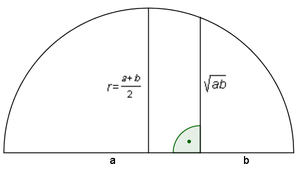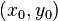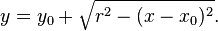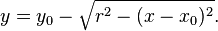# SemicircleIn mathematics (and more specifically geometry), a semicircle is a one-dimensional locus of points that forms half of a circle. The full arc of a semicircle always measures 180° (equivalently, π radians, or a half-turn). It has only one line of symmetry (reflection symmetry). In non-technical usage, the term "semicircle" is sometimes used to refer to a half-disk, which is a two-dimensional geometric shape that also includes the diameter segment from one end of the arc to the other as well as all the interior points.

By Thales' theorem, any triangle inscribed in a semicircle with a vertex at each of the endpoints of the semicircle and the third vertex elsewhere on the semicircle is a right triangle, with right angle at the third vertex.

All lines intersecting the semicircle perpendicularly are concurrent at the center of the circle containing the given semicircle.

## UsesA semicircle can be used to construct the arithmetic and geometric means of two lengths using straight-edge and compass. If we make a semicircle with a diameter of a+b, then the length of its radius is the arithmetic mean of a and b (since the radius is half of the diameter). The geometric mean can be found by dividing the diameter into two segments of lengths a and b, and then connecting their common endpoint to the semicircle with a segment perpendicular to the diameter. The length of the resulting segment is the geometric mean, which can be proved using the Pythagorean theorem. This can be used to accomplish quadrature of a rectangle (since a square whose sides are equal to the geometric mean of the sides of a rectangle has the same area as the rectangle), and thus of any figure for which we can construct a rectangle of equal area, such as any polygon (but not a circle).

## Equation

The equation of a semicircle with midpoint$(x_0,y_0)$ on the diameter between its endpoints and which is entirely concave from below is$y=y_0+\sqrt{r^2-(x-x_0)^2}.$

If it is entirely concave from above, the equation is$y=y_0-\sqrt{r^2-(x-x_0)^2}.$

## Arbelos

An arbelos is a region in the plane bounded by three semicircles connected at the corners, all on the same side of a straight line (the baseline) that contains their diameters.